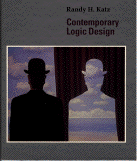# Contemporary Logic Design

Randy H. Katz
University of California
1993

1. Introduction
2. Two-Level Combinational Logic
3. Multilevel Combinational Logic
4. Programmable and Steering Logic
5. Arithmetic Circuits
6. Sequential Logic Design
7. Sequential Logic Case Studies
8. Finite State Machine Design
9. Finite State Machine Optimization
10. Finite State Machine Implementation
11. Computer Organization
12. Controller Implementation

Appendix A: Number Systems